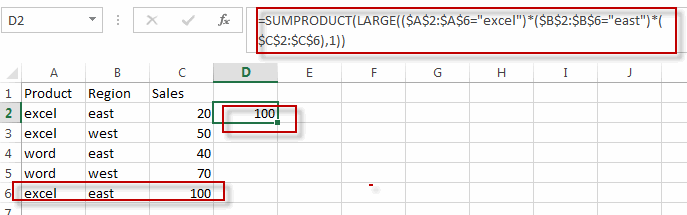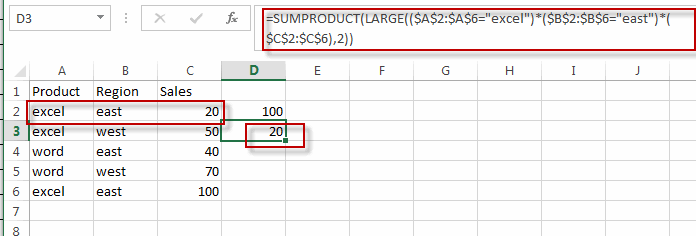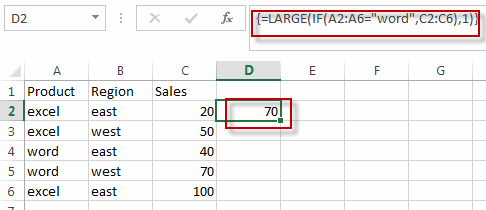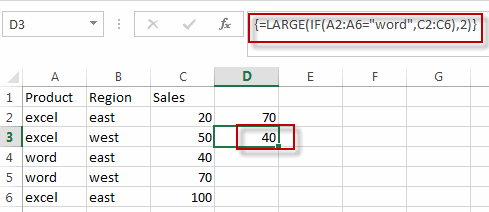# Find the Largest Value Based on Multiple Criteria

This post will guide you how to find the largest value based on one criteria in excel. How do I return the largest value based on multiple criteria in excel. How to find and return the largest value based on one criteria and multiple criteria with formula in excel.

## Find Largest Value with multiple Criteria

Assuming that you have a list of data that you want to find the largest value based on the product “excel” and the sales region “east”. You can create a new excel formula based on the SUMPRODUCT function and the LARGE function, just using the following formula:

`=SUMPRODUCT(LARGE((\$A\$2:\$A\$6="excel")*(\$B\$2:\$B\$6="east")*(\$C\$2:\$C\$6),1))`

You just need to type this formula into the formula box of cell D2, then press Enter key in your keyboard.This formula will find the first largest value based on the multiple criteria. And if you want to find the second largest value based on multiple criteria, just need to change “1” to “2”, just like this:

`=SUMPRODUCT(LARGE((\$A\$2:\$A\$6="excel")*(\$B\$2:\$B\$6="east")*(\$C\$2:\$C\$6),2))`## Find Largest Value with Only One Criteria

If you just want to find largest value with only one criteria, for example, you want to find the largest sales value based on the product “word”, then you can create a formula based on the LARGE function and the IF function. Just write down the following array formula as follows:

`=LARGE(IF(A2:A6="word",C2:C6),1)`

When you type this formula into the formula box of Cell D2, then press Ctrl +shift+ Enter keys together in your keyboard. Then the formula will become an array formula.This formula will get the first largest value based one criteria, and if you want to find the second or nth largest value based one criteria, you can change “1” to 2 or other number.

`=LARGE(IF(A2:A6="word",C2:C6),2)`### Related Functions

• Excel SUMPRODUCT function
The Excel SUMPRODUCT function multiplies corresponding components in the given one or more arrays or ranges, and returns the sum of those products.The syntax of the SUMPRODUCT function is as below:= SUMPRODUCT (array1,[array2],…)…
• Excel LARGE function
The Excel LARGE function returns the largest numeric value from the numbers that you provided. Or returns the largest value in the array. The syntax of the LARGE function is as below:= LARGE (array,nth)…
• Excel IF function
The Excel IF function perform a logical test to return one value if the condition is TRUE and return another value if the condition is FALSE. The IF function is a build-in function in Microsoft Excel and it is categorized as a Logical Function.The syntax of the IF function is as below:= IF (condition, [true_value], [false_value])….